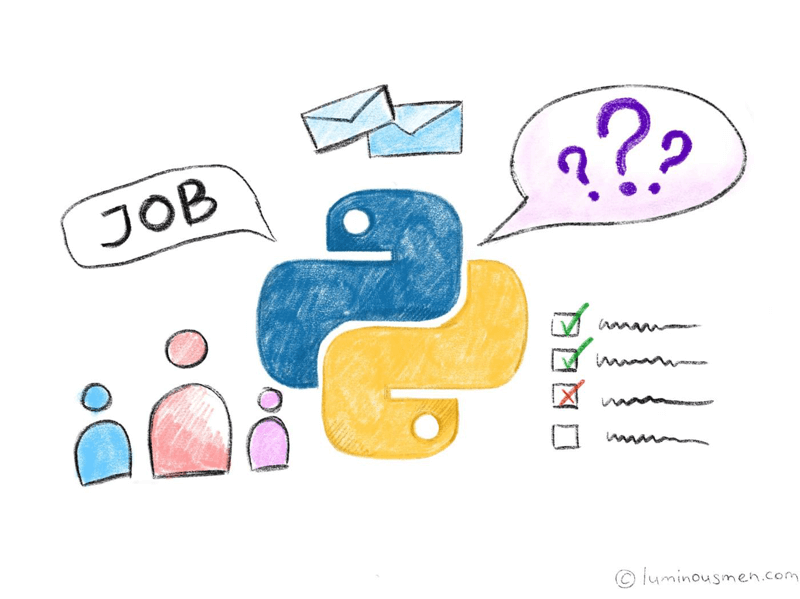# Python interview questions. Part II. MiddleI am starting a series of posts about my own base of interviewing questions. Of course, it is not full and it doesn't have any answers and maybe some of the questions seem weird or even stupid, but I think they are good for finding the right candidate for Python position.

## Questions

Q: What is the output of `-12 % 10`?

Q: What is the output of `-12 // 10`?

Q: What is the sequence of call operators in the expression `a * b * c`?

Q: Why shouldn't you make the default arguments an empty list?

Q: What `id()` function in Python is for?

Q: What is `yield` keyword is for?

Q: What are iterators in Python?

Q: What are generators in Python? Are they different from iterators?

Q: What is the difference between `__iter__` and `__next__`?

Q: How do you create a dictionary which can preserve the order of pairs?

Q: What is context manager? How are they different from `try ... finally`?

Q: What functions need to be overridden in class A in order for its instances to implement the context managers protocol?

Q: What is the synchronous code? What is the asynchronous code? How to write asynchronous code?

Q: What is `unittest` in Python?

Q: What is type checking? Do we have types in Python?

Q: How can you copy an object in Python?

Q: How memory is managed in Python?

Q: How the garbage collector works in Python? Describe Python's garbage collection mechanism in brief.

Q: How can you share global variables across modules?

Q: What is the attribute `__slots__`?

Q: What are metaclasses in Python?

Q: How to create a class without a class statement?

## Code involving questions

Q: What will be the output of the following code?

``````>>> list = ['a', 'b', 'c', 'd', 'e']
>>> print list[10:] # []
``````

Q: How can I reload a previously imported module? (we assume that the module is a file with `module.py`)

Q: What will be the output of the following code?

``````>>> a = [[]]*3
>>> a.append(1)
>>> print(a)  # [, , ]
``````

Q: What's wrong with this code?

``````def foo():
from .module import *
print(f"{bar()}")
``````

Q: The file is located in `/usr/lib/python/person.py`. The program starts as `python /usr/lib/python/person.py`. What is the output?

``````class Person:
def __init__(self, name):
__name__ = name

def getAge(self):
print(__name__)

p = Person("John")
p.getAge()
``````

Q: Write `timeit` decorator for measure time of function execution.

Q: Write repeater decorator that will catch errors several times(customizable).

Q: What's the output?

``````class parent:
def __init__(self, param):
self.v1 = param

class child:
def __init__(self, param):
self.v2 = param

obj = child(11)
print(obj.v1 + " " + obj.v2)
``````

Q: Add some code to make it work

``````class Repeater:
...
class RepeaterIterator:
...

repeater = Repeater("Hello")
for i in repeater:
print(i)  # hello
``````

Q: Write the code for getting unique values from list complex types.

Q: We have the following code with the unknown function f(). In f(), we do not want to use a return, instead, we may want to use a generator.

``````for x in f(5):
print x,
``````

The output looks like this:

``````0 1 8 27 64
``````

Write a function `f()` so that we can have the output above.

Q: What's the output?

``````x = [, ]
print(len(' '.join(list(map(str, x)))))
``````

Q: Write a program that prints the numbers from 1 to 20. But for multiples of three print “Fizz” instead of the number and for the multiples of five print “Buzz”. For numbers which are multiples of both three and five print “FizzBuzz”.

Q: Output? What is wrong? How to fix?

``````class Developer:
coffee_cups = 0

def __init__(self, name):
self.name = name
self.coffee_cups += 1

def speak(self):
print(f"I'm {self.name} and I've had {self.coffee_cups} cups of coffee today")

rover = Developer("Steve")
spot = Developer("Bob")
``````

Previous:

Python interview questions. Part I. Junior

Next:

Python interview questions. Part III. SeniorBuy me a coffee

More? Well, there you go: